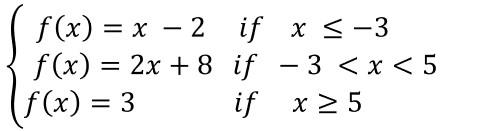Test Prep Plan - Take a practice test

Take this practice test to check your existing knowledge of the course material. We'll review your answers and create a Test Prep Plan for you based on your results.
How Test Prep Plans work
1
2Based on your results, we'll create a customized Test Prep Plan just for you!
3Study smarter
Study more effectively: skip concepts you already know and focus on what you still need to learn.

CLEP Precalculus: Study Guide & Test Prep Final Exam

Free Practice Test Instructions:

Choose your answer to the question and click 'Continue' to see how you did. Then click 'Next Question' to answer the next question. When you have completed the free practice test, click 'View Results' to see your results. Good luck!

Question 1 1. Solve for the value of x in the equation below: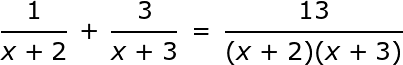Question 2 2. Solve for x in the equation below.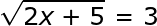Question 4 4. If f(x) = 2, what is the value of b?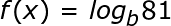Question 5 5. The graphs shown below are graphs of exponential functions. In the equation y = abx, what is a possible value for a to change the black graph into the blue graph?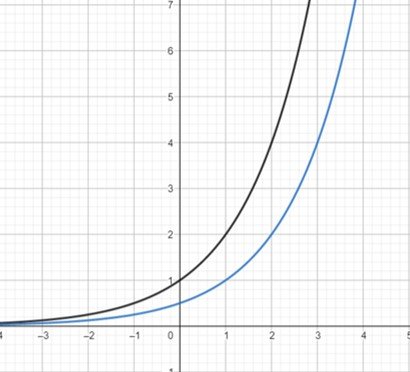Question 7 7. Solve for a.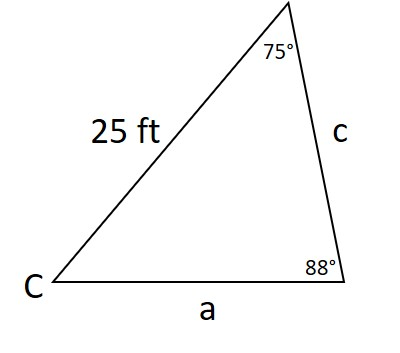Question 8 8. Solve for c.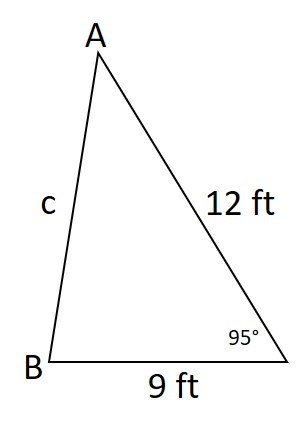Question 9 9. Identify the line of symmetry.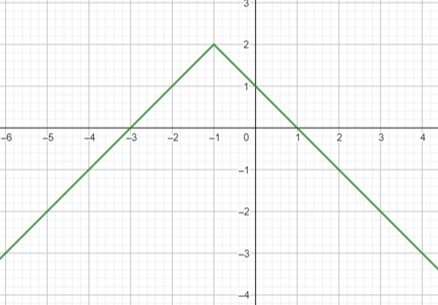Question 10 10. What is the range of the graph?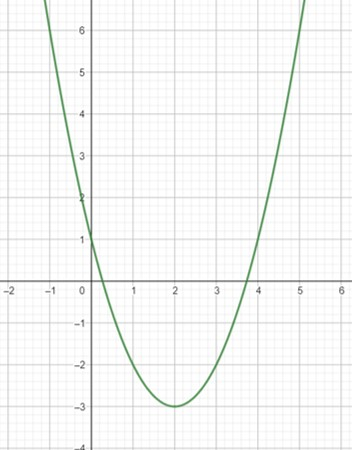Question 13 13. Find the domain of the graph.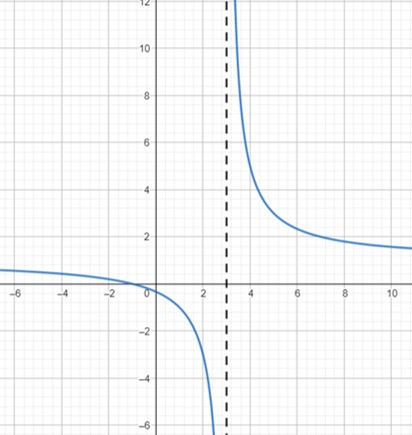Question 15 15. What is the value of f(x) when x = -3?